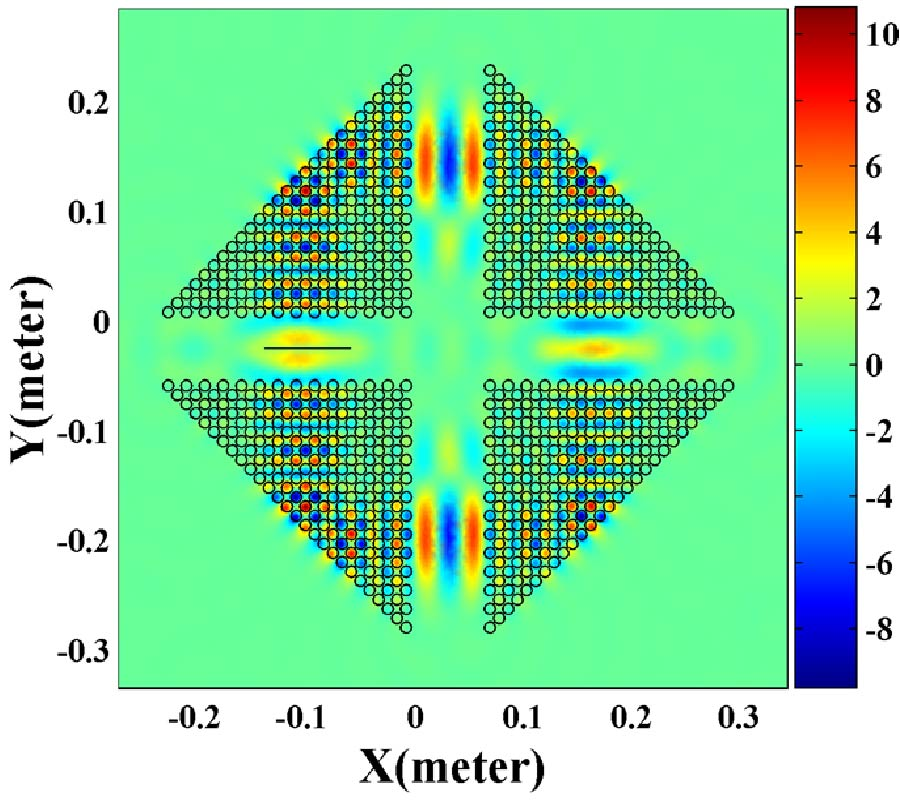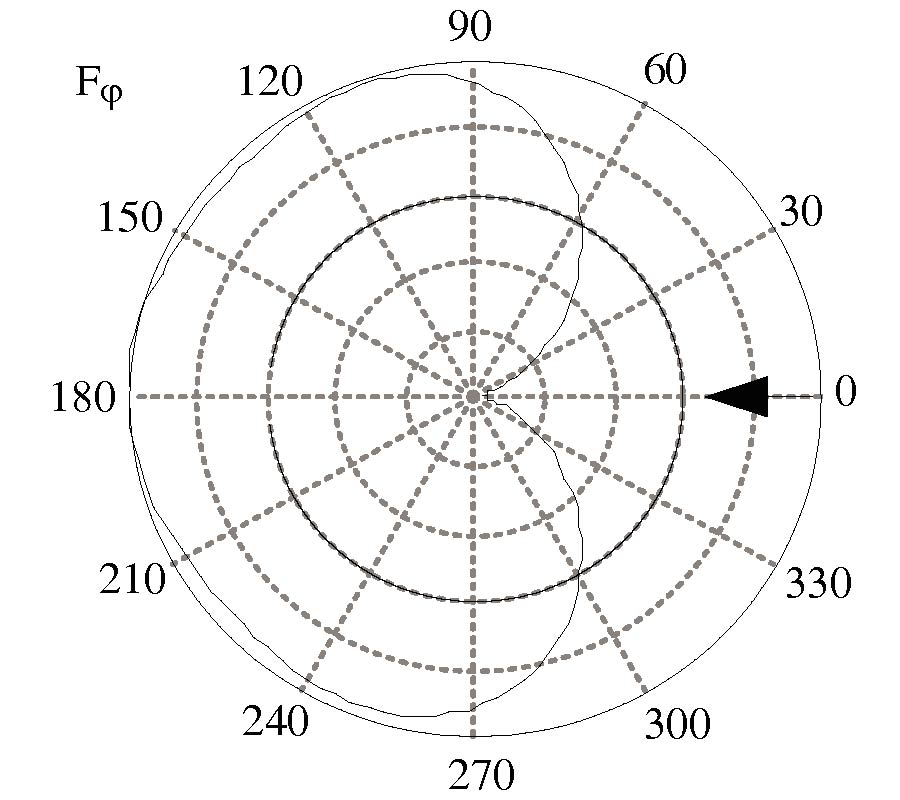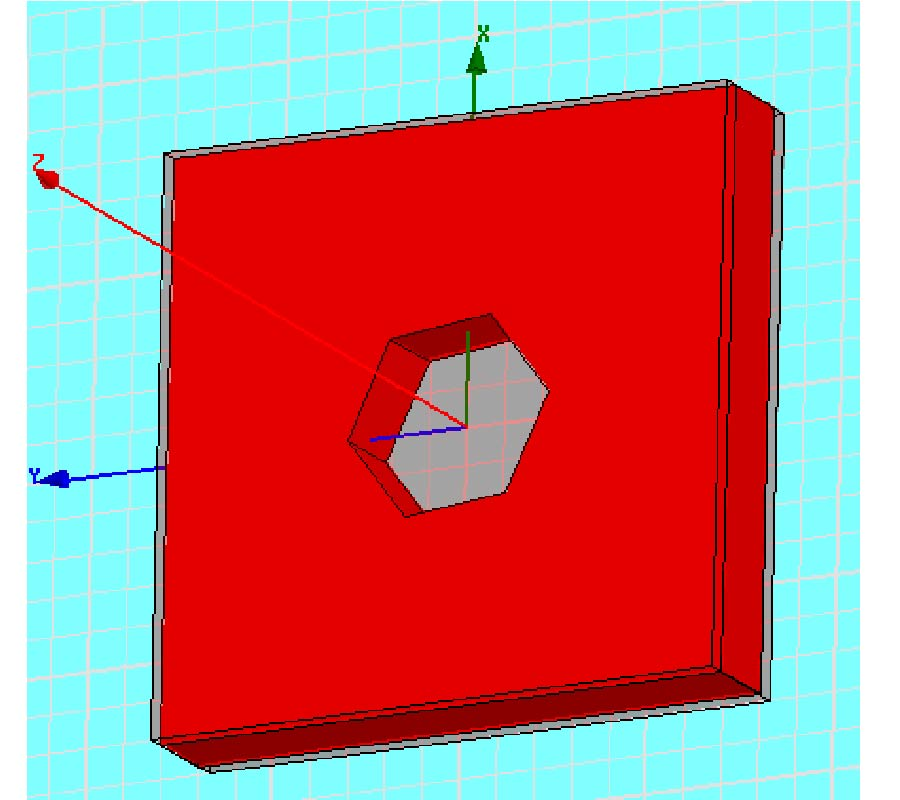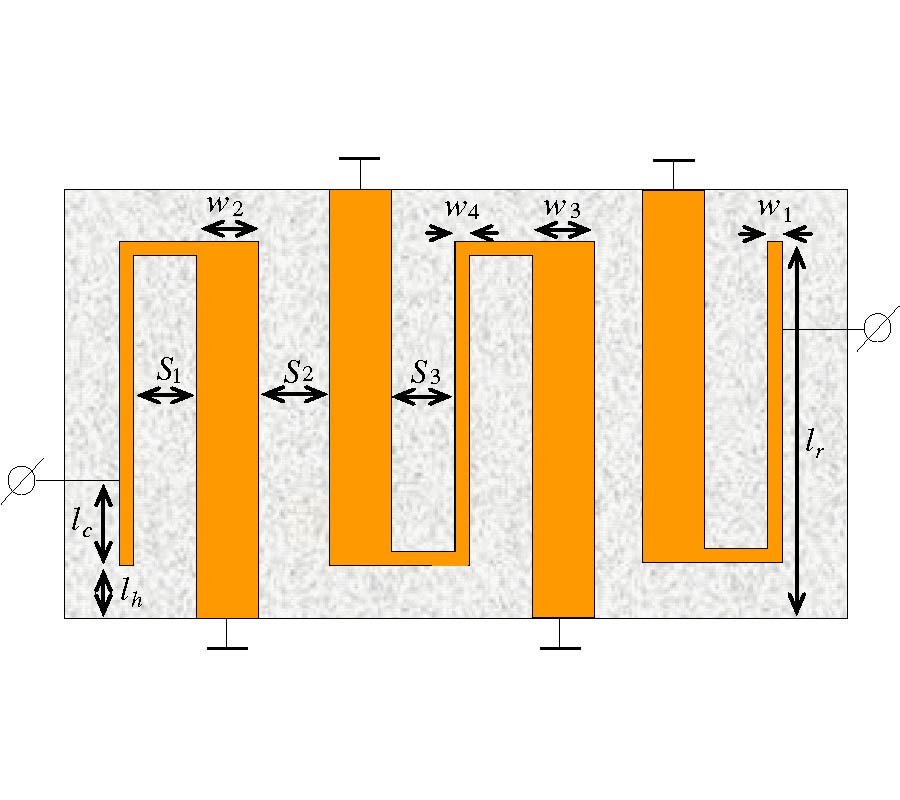ISSN: 1937-8726

# Vol. 12

Front:[PDF file] Back:[PDF file]
Latest Volume
All Volumes
All Issues

2010-07-08 PIER M Vol. 12, 247-257, 2010. doi:10.2528/PIERM10041302

###Cad Model to Compute the Input Impedance of an Equilateral Triangular Microstrip Patch Antenna with Radome

Manotosh Biswas and Anirban Mandal

A very simple and more efficient CAD model is proposed to demonstrate the effect on input impedance characteristics based on cavity model analysis for wide range of variations of superstrate parameters and feed locations of an equilateral triangular microstrip patch antenna having different side lengths. The computed values are compared with different theoretical and experimental values available in open literature, showing close agreement. A Maxwell's equation solver is also used to validate our model.

2010-06-02 PIER M Vol. 12, 229-245, 2010. doi:10.2528/PIERM10030704

###A Novel Phase Retrieval Approach for Electromagnetic Inverse Scattering Problem with Intensity-Only Data

Yin Xiang, Lianlin Li, and Fang Li

To measure the phase of signal with very high working frequency such as THz, and optics band is still a challenging problem. In this paper, based on the relationship between radiating current and measured intensity of electrical field a novel phase retrieval algorithm has been developed. As opposed to the existing approaches of phase retrieval where usually the Fourier coefficients of measured data will be firstly reconstructed, the proposed approach is to reconstruct the so-called radiating currents, with more physical meaning than the former. It has a much smaller number of freedoms of radiating current than that of measurements, which means that the obtained equations are over-determined. Thus one can efficiently model the intensity of measured electric field via the radiating part, and reconstruct it quickly and stably. The novelty is that this physical consideration 1) leads to efficiently avoiding false solutions due to the ill-posedness of phase retrieval problem, and 2) offers a good initial guess for inverse scattering based imaging algorisms. Importantly, a closed-form formulation of phase retrieve also has been derived when the intensity of incident wave is much stronger than one of the scattered wave, for example, for the weak scattering objects. Finally, several numerical experiments are provided to show the high performance of proposed algorithm.

2010-05-28 PIER M Vol. 12, 217-228, 2010. doi:10.2528/PIERM10042607

###Parallel MoM Solution of JMCFIE for Scattering by 3-D Electrically Large Dielectric Objects

Zhiwei Cui, Yiping Han, Qiang Xu, and Minglei Li

In this paper, we apply the parallel method of moments (MOM) to solve the Electric and Magnetic Current Combined Field Integral Equation (JMCFIE) for scattering by large, three-dimensional (3-D), arbitrarily shaped, homogeneous dielectric objects. We first derive the JMCFIE formulation which produces well-conditioned matrix equation when the MOM with Galerkin's type testing and Rao- Wilton-Glisson (RWG) functions is applied. We then develop a parallel conjugate gradient (CG) method on personal computer (PC) clusters using message passing interface (MPI) for solving the matrix equation obtained with JMCFIE. The matrix is decomposed by the row and stored in distributed memory of the node. Several numerical results are presented to demonstrate the accuracy and capability of the proposed method.

2010-05-28 PIER M Vol. 12, 205-216, 2010. doi:10.2528/PIERM10041402

###Three-Dimensional Electromagnetic Diffraction by a Slot System with Parallel Plane Dielectric Interfaces

An efficient method is presented for rigorous description of three-dimensional electromagnetic diffraction fields in slot systems containing several parallel plane interfaces between dielectrics and conductors. For such structures, the method employs the representation of spatial field components in terms of two complex scalar functions. They specify two field polarizations, which reflect and refract on all parallel dielectric interfaces independently, one from the other, which essentially simplify the total solution of diffraction problem. As an example, the application of eigen-function expansions and mode-matching technique solves the specific problem of three-dimensional diffraction of a plane electromagnetic wave by a slot in a thin conducting screen located ahead of a half-infinite dielectric.

2010-05-27 PIER M Vol. 12, 193-204, 2010. doi:10.2528/PIERM10040405

###On Developing Alternating Voltage Around a Rotating Circular Ring Under Plane Wave Excitation in the Presence of an Eccentrically Positioned Metallic Core

Constantinos Valagiannopoulos

Rotating coils constitute a type of electrical transformers used to produce alternating voltage pulses exploiting the phenomenon of electromagnetic induction. In this study, we investigate the influence of the electromagnetic scattering from a metallic obstacle located inside the moving component. In particular, a perfectly conducting spherical core is positioned eccentrically inside a thin circular ring, rotating around an arbitrary axis passing through its own center, under plane wave excitation. Methods and formulas implemented in scattering and induction problems have been utilized for the derivation of the developed potential difference around the loop. Several graphs of the voltage output versus the geometrical characteristics of the con guration, are shown and explained.

2010-05-20 PIER M Vol. 12, 181-192, 2010. doi:10.2528/PIERM10041002

###Investigation of Electromagnetic Shielding Rooms with Metal Cabinet and Aperture

In this paper, a fast and efficient method has been proposed to analyze the electromagnetic shielding rooms with electrical large sizes and arbitrary shapes. The ray-tracing method is used to predict the Shielding Effectiveness (SE) of the electromagnetic shielding rooms. The proposed method is based on speeding up the ray tracing algorithm. The performance of the proposed method is verified by a comprehensive example. The effect of additional metal cabinet in the shielding effectiveness of shielding room has been investigated. Also the position of it has been found optimally to produce a ``best" performance for the shielding room.

2010-05-10 PIER M Vol. 12, 165-180, 2010. doi:10.2528/PIERM10021102

###Numerical and Experimental Analysis of Electromagnetic Torque for Modular Toroidal Coil Applicable to Tokomak Reactors

Mohammad Reza Pahlavani, Abbas Shiri, and Abbas Shoulaie

A Modular Toroidal Coil (MTC) is composed of several solenoidal coils (SCs), which are connected in a series and distributed in the toroidal and symmetrical form. This paper presents analytical equations of mutual inductance and electromagnetic torque of the MTC applicable to Tokomak reactors. These equations are based on those formulated by Neumann. The numerical analysis of the integrations resulting from these equations is solved using the extended three-point Gaussian algorithm. The results obtained from the numerical simulation agree with the empirical results, the experimental results, and the virtual work theorem, which indicates the reliability of the presented equations. The behavior of the mutual inductance of the coil shows that the maximum stored energy is obtained when the electromagnetic torque is zero, and vise versa.

2010-05-03 PIER M Vol. 12, 155-164, 2010. doi:10.2528/PIERM10032405

###Coupling Between an Irradiated Aperture and Monopole Antennas into a Complex Enclosure

François Caudron, Achour Ouslimani, Rene Vezinet, Abed-elhak Kasbari, Jean-Luc Lavergne, and Jean-Sébastien Borrod

The coupling between an irradiated aperture and a monopole antenna into a complex enclosure is investigated. The aperture is realized at the one side of the enclosure and the monopole antenna at the other side. The proposed study uses Babinet's principle to extend the Random Coupling Model to determine the radiation impedance of apertures. An experimental study is carried out using a computer box as an enclosure. A high intensity external electromagnetic radiation is applied to the aperture. The induced voltages is measured along the monopole antenna. The simulated probability levels of the induced voltages agree well with the experimental ones.

2010-05-03 PIER M Vol. 12, 143-153, 2010. doi:10.2528/PIERM10033104

###New Formula for the Reflection Coefficient of an Open-Ended Rectangular Waveguide with OR Without an Infinite Flange

Jong-Heon Kim, Bayanmunkh Enkhbayar, Jae-Hoon Bang, Bierng-Chearl Ahn, and Eun-Jong Cha

New formulas are presented for the reflection coefficient at the open end of a rectangular waveguide radiating into air including the effect of wall thickness or flange. Existing formulas require significant amount of numerical calculations and do not cover the practical range of waveguide dimensions. Reflection coefficients of open-ended standard waveguides are simulated using commercial electromagnetic software and curve-fitted to derive new formulas. Proposed formulas include the effects of waveguide wall thickness and broad-to-narrow wall aspect ratio. The accuracy of proposed formulas is compared with existing analytical, numerical and experimental results.

2010-05-03 PIER M Vol. 12, 131-141, 2010. doi:10.2528/PIERM10040905

###Path Loss Modeling for Near-Ground VHF Radio-Wave Propagation through Forests with Tree-Canopy Reflection Effect

Yu Song Meng, Yee Hui Lee, and Boon Chong Ng

This paper presents a new methodology to model the near-ground short-range propagation loss in forested areas at the VHF and the UHF band. The path loss modeling is performed by an integration of the foliage induced effect and the effect from the radio-wave reflection/reflections. The analysis shows that the reflection from the dense tree canopy is important for short-range propagation at the VHF band and therefore, cannot be ignored. When taking into consideration the contribution from the possible tree-canopy reflection, the modeled path loss is reduced by more than 15 dB in the VHF band. A good agreement of the modeled path loss with the measured loss in tropical forested areas is achieved.

2010-04-29 PIER M Vol. 12, 115-130, 2010. doi:10.2528/PIERM10012604

###Analysis of Self-Collimation Based Cavity Resonator Formed by Photonic Crystal

Natesan Yogesh and Venkatachalam Subramanian

The self-collimation effect in photonic crystal is used for the realization of open cavity resonator formed by photonic prisms in a four-port arrangement. The confinement, field enhancement and energy storage capabilities of the proposed cavity are explored in this paper. The effect of dielectric losses included in the system and role of the position of line source in the confinement effect of the cavity are brought out. Decay of short Gaussian pulse placed inside the cavity is analyzed through finite-difference time-domain studies. Due to the high confinement and divergence less beam propagation, utility of the proposed cavity for rotational gyroscope application is also revealed.

2010-04-29 PIER M Vol. 12, 107-113, 2010. doi:10.2528/PIERM10031401

###Self-Consistent Approach to the Electrodynamic Analysis of the Chiral Structures

Vyacheslav Alexandrovich Neganov, Dmitry Petrovich Tabakov, and Ivan Mikhailovich Gradinar

Rigorous electrodynamic analysis is proposed to estimate the surface current density on the perfectly conducting chiral elements in the diffraction problems. It is reduced to the solution of the integral singular equations. The diffraction of plane electromagnetic wave on the cylindrical open ring is considered as an example.

2010-04-27 PIER M Vol. 12, 95-105, 2010. doi:10.2528/PIERM10030306

###Characterization of the Regular Polygonal Waveguide for the RF EM Shielding Application

Blaise Ravelo and Belahcene Mazari

This article presents a theoretical characterization of the regular polygonal waveguide (RPW) having n-sides. Based on the symmetrical circular symmetry of the RPW and the circular waveguide (CW), the analogy between the electromagnetic (EM) behaviors of these to waveguide (WG) is established. After a brief recall about the state of the art concerning the WG engineering and its application, we introduce a basic theory of the WG presenting a regular polygonal cross-section with n-sides. By considering, the fundamental mode TE11, we develop the main mathematical formulas summarizing the different characteristics (cut-off frequency, fc, propagating constant, k11 and S-parameters) appropriated to any RPW in function of its physical parameters (number of sides, n, diameter, D and height, h). In order to verify the validation of the developed analytical expressions, comparisons between the HFSS simulated and theoretical dispersion diagrams of regular pentagonal (n=5), hexagonal (n=6), heptagonal (n=5) metallic (copper) WG with for example, 50 mm outer diameter are presented. So, it was demonstrated that very good correlation between the theoretical predictions (fc(n), k11(n)) is found with a relative error less than 1%. As application of the present study in terms of EM wave shielding, simulation of metallic wall with hexagonal aperture is also performed. Finally, discussion about the future work is drawn in conclusion.

2010-04-27 PIER M Vol. 12, 81-93, 2010. doi:10.2528/PIERM10031102

###On the Distribution of Current on an Open Tape Helix

Natarajan Kalyanasundaram, Gnanamoorthi Babu, and Rahul Tulsian

The approximate distribution of the current density induced on the tape surface by guided electromagnetic waves supported by an infinite open tape helix is estimated from an exact solution of a homogenous boundary value problem for Maxwell's equations. It is shown that the magnitude of the surface current density component perpendicular to the winding direction is at least three orders of magnitude smaller than that of the surface current density component parallel to the winding direction everywhere on the tape surface. Also, the magnitude and phase distribution for the surface current density components parallel and perpendicular to the winding direction are seen to be nearly uniform at all frequencies corresponding to real values of the propagation constant.

2010-04-23 PIER M Vol. 12, 67-79, 2010. doi:10.2528/PIERM10031505

###Effective Plasma Frequency for Two- Dimensional Metallic Photonic Crystals

Khee Lam Low, Mohd Zubir Mat Jafri, and Sohail A. Khan

Generalized band structure equation for photonic crystals which containing dielectric rods in metals medium was derived by using the plane wave expansion method. From the band structure, we can study band gap of photonic crystals in both E and H polarizations. Since metals are frequency-dependant materials, modification needs to be done on the plane wave expansion equation to calculate the metallic photonic crystals containing dielectric constant rods. To ease the calculation, simple Drude model for metals are used. In this model, the equation is without damping constant. We have plotted the band structure for photonic crystals in metals medium. Then, we studied the ffective plasma frequency of the structure from the band graph in E polarization mode (TM). We found that effective plasma frequency can be tailored as we want. Detailed results are presented with different sizes of radius. Comparison is made for different background materials.

2010-04-19 PIER M Vol. 12, 51-65, 2010. doi:10.2528/PIERM09122901

###Shift-Operator FDTD Method for Anisotropic Plasma in Kdb Coordinates System

Lai-Xuan Ma, Hou Zhang, Hong-Xing Zheng, and Chen-Xin Zhang

Electromagnetic (EM) problem model for anisotropic plasma in kDB coordinates system is set up. And the model includes almost all the respects of EM-problems for anisotropic plasma. Based on shift-operator finite difference time-domain (SO-FDTD) method, Maxwell equations and EM-field constitutive equations are solved and discrete difference scheme of each EM-field component is obtained. Then the propagation characteristics of eigen wave are expressed by the two components of electric displacement vector as well. Lastly, three typical examples are calculated by SO-FDTD method, and the results verify the effectiveness and exactness of SO-FDTD method in kDB coordinates system.

2010-04-15 PIER M Vol. 12, 39-50, 2010. doi:10.2528/PIERM10012211

###Electromagnetic Bandgap Analysis of 1D Magnetized Ppc with Oblique Incidence

Lixia Yang, Yingtao Xie, Pingping Yu, and Gang Wang

The modified finite-difference time-domain (M-FDTD) method is proposed to analysis electromagnetic bandgap of 1D layered anisotropic plasma photonic crystal(PPC) under the situa-tion of the EM wave oblique incidence. The presence of it avoids the usage of two-dimensional FDTD iterative and greatly improving the computational efficiency. By the algorithm, the reflec-tion coefficients of electromagnetic waves with different incidence angles are computed, and compare the results with the analytical solution. The results show that the method of the accuracy and effectiveness. Finally, the algorithm is applied to calculate electromagnetic bandgap charater-istics of PPC with the different incident angles, and their reflection coefficients under the condi-tion of the different incident angles are analyzed.

2010-04-12 PIER M Vol. 12, 29-38, 2010. doi:10.2528/PIERM10011902

###A New Hybrid mm /Compact 2-D Fdfd Method for Rectangular Ridged Waveguide Discontinuities

Wei Zhao, Yong-Jiu Zhao, Hong-Wei Deng, Duanwei Zhang, and Bing Liu

A hybrid mode-matching/compact 2-D finite-difference frequency-domain (MM/compact 2-D FDFD) method is proposed for the analysis of rectangular ridged waveguide discontinuities. In order to apply MM technique, mode spectrum of the ridged waveguide is determined by an improved compact 2-D FDFD method with only two transverse field components at the cutoff frequencies which lead to two independent sets of real symmetric eigenvalue problems for TE and TM modes. Solving these two separate eigenvalue equations, cutoff wave numbers and discrete mode field functions can be obtained respectively from eigenvalues and eigenvectors. Finally, the generalized scattering matrix (GSM) of the rectangular-ridged waveguide step discontinuity can be easily calculated through the transverse field matching procedure. The method is demonstrated at the examples of two waveguide structures, and results are shown to be in excellent agreement with those by the commercial CAD software HFSS.

2010-04-08 PIER M Vol. 12, 17-28, 2010. doi:10.2528/PIERM10012507

###Synthesis and Investigation of Three-Section Microstrip Filter on Folded Dual-Mode Stepped-Impedance Resonators

Ivan A. Dovbysh and Vladimir V. Tyurnev

A new structure of an ultra-wide bandpass filter is considered. An intelligence method of optimization is applied first for synthesis of three-section filter on dual-mode resonators. An area of fractional bandwidth values and values of dielectric constant of the substrate, where synthesis problem for the filter has a solution, is determined theoretically. Experimental results show good agreement with simulated values.

2010-04-08 PIER M Vol. 12, 1-15, 2010. doi:10.2528/PIERM10022003

###A Cell-Vertex Finite Volume Time Domain Method for Electromagnetic Scattering

Narendra Deore and Avijit Chatterjee

A cell-vertex based finite volume scheme is used to solve the time-dependentMaxwell's equations and predict electromagnetic scattering from perfectly conducting bodies. The scheme is based on the cell-vertex finite volume integration method, originally proposed by Ni, for solution of the two dimensional unsteady Euler equations of gas dynamics. The resulting solution is second-order accurate in space and time, and requires cell based fluctuations to be appropriately distributed to the state vector stored at cell vertices at each time step. Results are presented for two-dimensional canonical shapes and complex three dimensional geometries. Unlike in gas dynamics, no user defined numerical damping is required in this novel cell-vertex based finite volume integration scheme when applied to the time-domain Maxwell's equations.